VII. FACTORISATION OVER C-, R- AND J-FIELDS

Use the factor theorem to show (1-4) that:

1.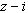is a factor of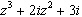2.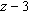is a factor of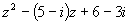3.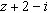is a factor of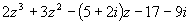4.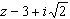is a factor of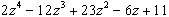5. Given that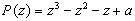, find the value of a if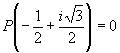6. Given that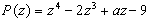, find the value of a if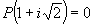.

7. Given that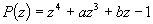, find the values of a and b if i and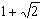are zeros of P(x).

8. Given that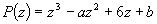, find the values of a and b if P(2)=0 and P(1-i)=0.

Complete factorise the following (9-24):

9.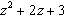over C

10.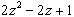over C

11.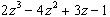over (i) J (ii) C

12.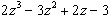over C.

13.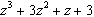over C.

14.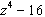over (i) J and (ii) C.

15.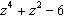over (i) Q (ii) R (iii) C.

16.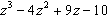over (i) R (ii) C

17.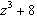over (i) R (ii) C.

18.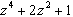over C.

19.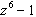over (i) R (ii) C.

20.over (i) R (ii) C.

21.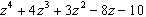over (i) R (ii) C given that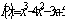is one linear factor.

22.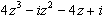over C.

23.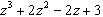over (i) R (ii) C.

24.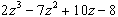over C.

Find z in (25-31);

25.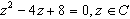26.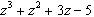if (i)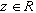(ii)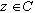27.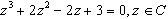28.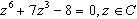29.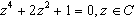30.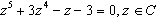31.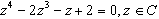32. Find the values of the real numbers a and b , such that 1+i is a root of the equation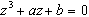Find z in 33-39:

33.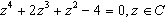34.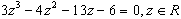35.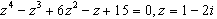36.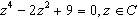37.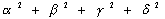38.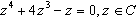39.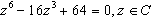Write down an equation of the lowest possible degree with (i) complex coefficients, (ii) rational coefficients and having the following among its roots:

40.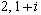41.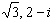42.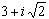,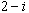43.of multiplicity 2.

44.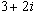45.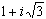Find and ploton the complex plane (46-55):

46. The square root of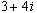47. The square root of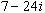48.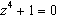49.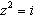50.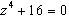51. The cube root of 64.

52. The fourth root of 8. 53. The sixth root of -1

54. The sixth root of 64. 55. The cube root of -8.

56. Find real values of a for which ai is a solution of the polynomial equation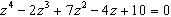57. Find the values which the real numbers a and b must take for z=1 to be a root of the complex equation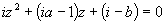58. If 1,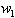, and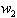are the cube roots of unity, prove that (i)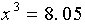, (ii)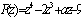and (iii)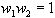.

59. If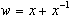, prove that

(i)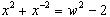(ii)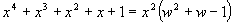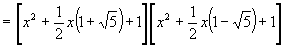Show that the roots of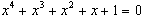are the four complex roots of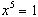. Deduce that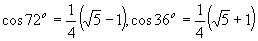60. Find (a) the three roots of the equation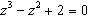(b) the remainder whenis divided by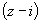61. Find the real numbers k such that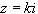is a root of the equation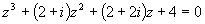. Hence or otherwise find the three roots of the equation.

62. Solve the following equations using a calculus method.

(a)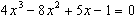given that it has a root of multiplicity 2.

(b)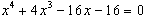given that it has a root of multiplicity 3.